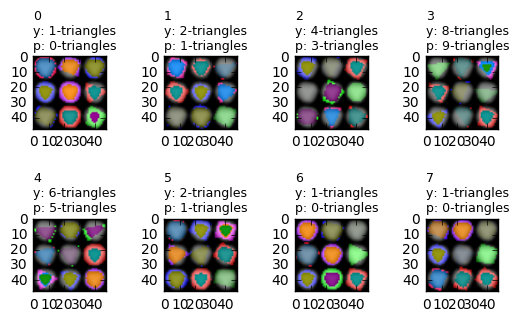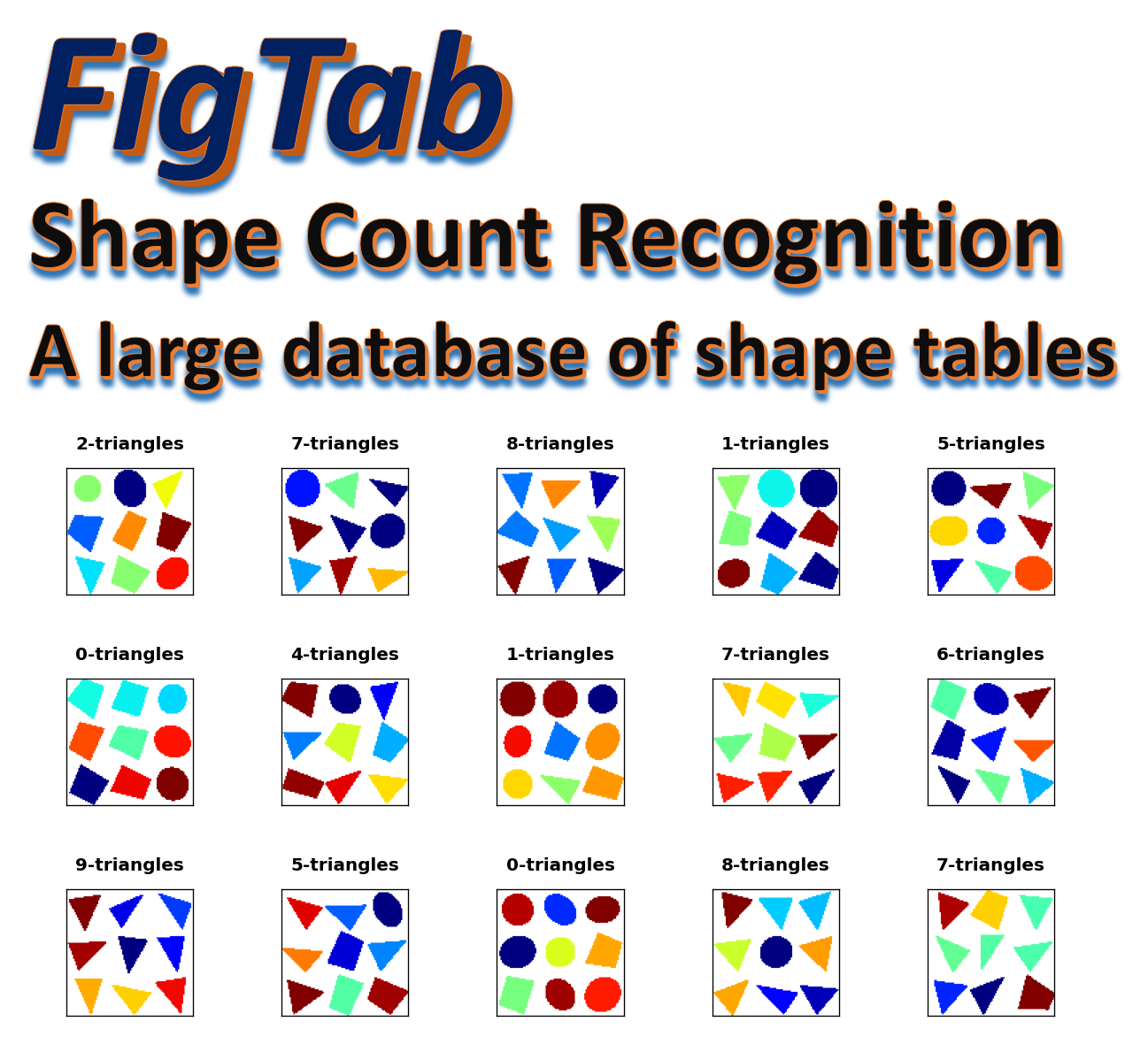FigTab is a data set of 48x48x3 pixel images of geometrical shapes. Each image consists of a 3x3 grid of 9 simple geometrical figures (ellipse, triangle, quadrilateral).

They were generated by an automatic Python script with randomization on shapes. We used 48x48x3 pixels images to make it possible for standard pc systems to run the exercises in this notebook (a modest graphics card is required though). It is intented to serve as a simple and clean data set for preliminary excursions, tutorials, or course projects in deep learning courses.

It is also intended to serve as a simple data set for benchmarking deep learning libraries and deep learning hardware (like GPU systems).

The simplest recognition task related to such data set is for example to count the number of occurrences of a particular shape in each image. In this notebook we will try to build an efficient neural network for detecting the exact number of triangles in each image. We will therfore have 10 categories which we assign the following names:

0: 0-triangles
1: 1-triangles
2: 2-triangles
3: 3-triangles
4: 4-triangles
5: 5-triangles
6: 6-triangles
7: 7-triangles
8: 8-triangles
9: 9-triangles



Some examples of this categories can be seen in the above diagram. Our neural network will accept a 48x48x3 pixel color image as input and will output the exact number of triangles this image has.

One can think of other recognition tasks, such as detecting all images that have the same shape along one of the diagonals, or in one row, etc. It would be intereseting to see if their solution requires the same effort or not? Of course, we leave these challenges as exercises and course projects for our dear students :-)

The FigTab data set consists of 2 HDF5 files, each contains 50,000 48x48x3 pixel color images. All images are unique, there are no duplications.

We also supply two smaller data sets for training and validation, since the above data sets are too large. The "train.h5" contains 10,000 samples (1000 from each class), and the "test.h5" data set contains 5000 samples (500 from each class):

You will need to install the h5py Python module. Reading and writing HDF5 files can be easily learned from the following tutorial: https://www.getdatajoy.com/learn/Read_and_Write_HDF5_from_Python

## Prerequisites¶

The code for this IPython notebook was tested on Windows 10, Python 2.7/3.5 with keras, numpy, matplotlib and jupyter. The deep learning hardware we used was an NVIDIA GPU (GeForce GTX950) with cuDNN version 5103. Of course, it can also be run on CPU but it will be significantly slower (not recommended).

To run the code in this notebook, you'll also need to download a few private libraries libraries which we use in other examples of this course:

Here are the Python modules and basic definitions we need for an example of how to use the figtab data set

In :
from keras.models import Sequential, load_model
from keras.layers import Dense, Dropout, Activation, Flatten
from keras.layers import Convolution2D, MaxPooling2D, AveragePooling2D, ZeroPadding2D
from keras.optimizers import SGD
from keras.constraints import maxnorm
from keras.utils import np_utils
from keras.utils.visualize_util import plot
from keras.layers.noise import GaussianNoise
from keras.preprocessing.image import ImageDataGenerator
import matplotlib.pyplot as plt
from kerutils import *
from imgutils import *
from matplotlib import rcParams
rcParams['figure.figsize'] = 10,7
%matplotlib inline

class_name = {
0: '0-triangles',
1: '1-triangles',
2: '2-triangles',
3: '3-triangles',
4: '4-triangles',
5: '5-triangles',
6: '6-triangles',
7: '7-triangles',
8: '8-triangles',
9: '9-triangles',
}

nb_classes = len(class_name)  # 10
classes = range(nb_classes)

Using Theano backend.
DEBUG: nvcc STDOUT mod.cu
Creating library C:/Users/samy/AppData/Local/Theano/compiledir_Windows-10-10.0.14393-Intel64_Family_6_Model_94_Stepping_3_GenuineIntel-2.7.11-64/tmp3m0_em/265abc51f7c376c224983485238ff1a5.lib and object C:/Users/samy/AppData/Local/Theano/compiledir_Windows-10-10.0.14393-Intel64_Family_6_Model_94_Stepping_3_GenuineIntel-2.7.11-64/tmp3m0_em/265abc51f7c376c224983485238ff1a5.exp

Using gpu device 0: GeForce GTX 950 (CNMeM is enabled with initial size: 80.0% of memory, cuDNN 5103)
c:\anaconda2\lib\site-packages\theano\sandbox\cuda\__init__.py:600: UserWarning: Your cuDNN version is more recent than the one Theano officially supports. If you see any problems, try updating Theano or downgrading cuDNN to version 5.
warnings.warn(warn)

In :
# These are css/html styles for good looking ipython notebooks
from IPython.core.display import HTML
HTML('<style>{}</style>'.format(css));


## Load training and test data¶

The imgutils module contains a utility load_data for loading HDF5 files to memory (as Numpy arrays). This method accepts the names of your training and validation data set files, and it returns the following six Numpy arrays:

1. X_train: an array of 8000 images whose shape is 8000x48x48.
2. y_train: a one dimensional array of 8000 integers representing the class of each image in X_train.
3. Y_train: an 8000 array of one-hot vectors needed for Keras model. For more details see: http://stackoverflow.com/questions/29831489/numpy-1-hot-array
4. X_test: an array of 1000 validation images (1000x48x48)
5. y_test: validation class array
6. Y_test: one-hot vectors for the validation samples

It should be noted that in additional to reading the images from the HDF5 file, the load_data method also performs some normalization of the image data like scaling it to a unit interval and centering it around the mean value. You can control these actions by additional optional options of this command. Please look at the source code to learn more.

In :
X_train, y_train, Y_train, X_test, y_test, Y_test = load_data('train.h5', 'test.h5')

print('X_train shape:', X_train.shape)
print(X_train.shape, 'training samples')
print(X_test.shape, 'validation samples')

Loading training data set: train.h5
Total num images in file: 10000
Time: 3.50 seconds
Total num images in file: 5000
Time: 1.95 seconds
10000 training samples
5000 validation samples
Image shape: (48L, 48L, 3L)
X_train shape: (10000L, 48L, 48L, 3L)
10000 training samples
5000 validation samples


The original data of each image is a 48x48x3 matrix of integers from 0 to 255. We need to scale it down to floats in the unit interval. This done automatically by the above load_data method, which applies the data_normalization procedure. Look at the imgutils module for more details.

Let's also write two small utilities for drawing samples of images, so we can inspect our results visually. We need to pull our images from the HDF5 files and not from the X_train and X_test arrays, since they were normalized and have significantly changed. Here is a method for extracting image number i from an HDF5 file:

In :
def get_img(hfile, i):
f = h5py.File(hfile,'r')
img = np.array(f.get('img_' + str(i)))
cls = f.get('cls_' + str(i)).value
f.close()
return img, cls

In :
def draw_image(hfile, i):
img, cls = get_img(hfile, i)
plt.imshow(img, cmap='jet')
plt.title(class_name[cls], fontsize=15, fontweight='bold', y=1.05)
plt.show()


Let's draw image 18 in the X_train array as example

In :
draw_image("train.h5", 18)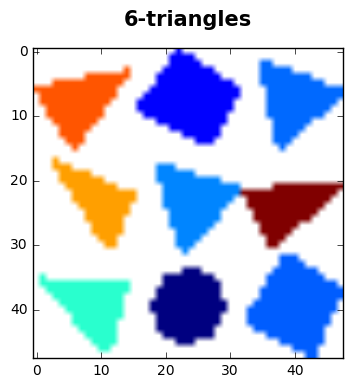Sometimes we want to inspect a larger group of images in parallel, so we also provide a method for drawing a grid of consecutive images.

In :
def draw_sample(hfile, n, rows=4, cols=4, imfile=None, fontsize=9):
for i in range(0, rows*cols):
ax = plt.subplot(rows, cols, i+1)
img, cls = get_img(hfile, n+i+1)
plt.imshow(img, cmap='jet')
plt.title(class_name[cls], fontsize=fontsize, y=1.08)
ax.get_xaxis().set_ticks([])
ax.get_yaxis().set_ticks([])

In :
draw_sample("train.h5", 400, 3, 5)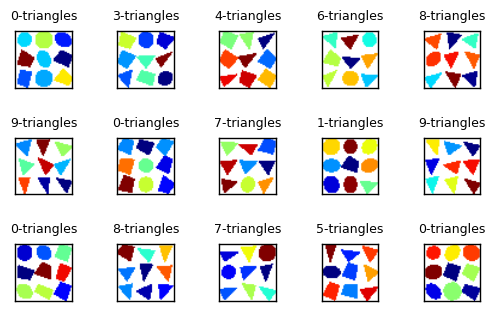# Building A Neural Network for FigTab¶

We will start with a simple Keras model which combines one Convolution2D layer with two Dense layers. Although simple in terms of code, it is too expensive in terms of computation and hardware, as it contains 70 million parameters! This is way too much and should be avoided in general. However, we want to experiment with the common use of Dense layers and see why they are not good for image processing. In general, Dense layers should be avoided as much as possible when dealing with image data. The general practice is to use Convolution and Pooling layers. These two types of layers are explained in more detail in the following two articles, which we recommend to read before you approach the following code:

## Lets Train Model 1¶

We now define our first model for the recognizing FigTab shapes. Note that unlike the common practice, we decided to use the SReLU activation method instead of the more popular relu activation. We did several test with relu but SReLU seems to be more appropriate for FigTab. One of the amazing facts about SReLU is that it adapts itself during the learning process and not a constant function as other activations. You may read more about it in the following papers:

In :
nb_epoch = 100
batch_size = 32
input_shape = X_train.shape[1:]

model = Sequential(name="model_1")

print(model.summary())
save_model_summary(model, "model_1_summary.txt")
write_file("model_1.json", model.to_json())
fmon = FitMonitor(thresh=0.09, minacc=0.999, filename="model_1_autosave.h5")

hist = model.fit(
X_train,
Y_train,
batch_size=batch_size,
nb_epoch=nb_epoch,
shuffle=True,
validation_data=(X_test, Y_test),
verbose=0,
callbacks = [fmon]
)

model_file = "model_1.h5"
print("Saving model to:", model_file)
model.save(model_file)
plot(model, to_file="model_1_scheme.png", show_layer_names=False, show_shapes=True)

show_scores(model, hist, X_train, Y_train, X_test, Y_test)

____________________________________________________________________________________________________
Layer (type)                     Output Shape          Param #     Connected to
====================================================================================================
convolution2d_1 (Convolution2D)  (None, 46L, 46L, 64)  1792        convolution2d_input_1
____________________________________________________________________________________________________
srelu_1 (SReLU)                  (None, 46L, 46L, 64)  541696      convolution2d_1
____________________________________________________________________________________________________
flatten_1 (Flatten)              (None, 135424)        0           srelu_1
____________________________________________________________________________________________________
dense_1 (Dense)                  (None, 512)           69337600    flatten_1
____________________________________________________________________________________________________
srelu_2 (SReLU)                  (None, 512)           2048        dense_1
____________________________________________________________________________________________________
dropout_1 (Dropout)              (None, 512)           0           srelu_2
____________________________________________________________________________________________________
dense_2 (Dense)                  (None, 256)           131328      dropout_1
____________________________________________________________________________________________________
srelu_3 (SReLU)                  (None, 256)           1024        dense_2
____________________________________________________________________________________________________
dropout_2 (Dropout)              (None, 256)           0           srelu_3
____________________________________________________________________________________________________
dense_3 (Dense)                  (None, 10)            2570        dropout_2
____________________________________________________________________________________________________
activation_1 (Activation)        (None, 10)            0           dense_3
====================================================================================================
Total params: 70,018,058
Trainable params: 70,018,058
Non-trainable params: 0
____________________________________________________________________________________________________
None
Train begin: 2017-01-06 01:35:22
Stop file: stop_training_file.keras (create this file to stop training gracefully)
Pause file: pause_training_file.keras (create this file to pause training and view graphs)
batch_size = 32
do_validation = True
metrics = ['loss', 'acc', 'val_loss', 'val_acc']
nb_epoch = 100
nb_sample = 10000
verbose = 0
.....05% epoch=5, acc=0.869100, loss=0.348462, val_acc=0.878200, val_loss=0.323298, time=0.058 hours
.....10% epoch=10, acc=0.923000, loss=0.218245, val_acc=0.902400, val_loss=0.287723, time=0.107 hours
.....15% epoch=15, acc=0.935100, loss=0.187559, val_acc=0.890400, val_loss=0.358969, time=0.156 hours
.....20% epoch=20, acc=0.953600, loss=0.144756, val_acc=0.892800, val_loss=0.367368, time=0.205 hours
.....25% epoch=25, acc=0.954900, loss=0.152405, val_acc=0.914800, val_loss=0.305429, time=0.254 hours
.....30% epoch=30, acc=0.964100, loss=0.113829, val_acc=0.917600, val_loss=0.308139, time=0.302 hours
.....35% epoch=35, acc=0.965000, loss=0.116226, val_acc=0.878600, val_loss=0.574214, time=0.351 hours
.....40% epoch=40, acc=0.970100, loss=0.097011, val_acc=0.884000, val_loss=0.516352, time=0.400 hours
.....45% epoch=45, acc=0.973100, loss=0.105366, val_acc=0.927000, val_loss=0.296137, time=0.449 hours
.....50% epoch=50, acc=0.975800, loss=0.084747, val_acc=0.912400, val_loss=0.388649, time=0.497 hours
.....55% epoch=55, acc=0.982400, loss=0.056027, val_acc=0.914200, val_loss=0.388883, time=0.546 hours
.....60% epoch=60, acc=0.981400, loss=0.070554, val_acc=0.905400, val_loss=0.436575, time=0.595 hours
.....65% epoch=65, acc=0.978400, loss=0.084123, val_acc=0.912400, val_loss=0.324411, time=0.644 hours
.....70% epoch=70, acc=0.986900, loss=0.045814, val_acc=0.890600, val_loss=0.522754, time=0.692 hours
.....75% epoch=75, acc=0.983200, loss=0.074486, val_acc=0.926200, val_loss=0.287778, time=0.741 hours
.....80% epoch=80, acc=0.978700, loss=0.076641, val_acc=0.912200, val_loss=0.388481, time=0.789 hours
.....85% epoch=85, acc=0.989900, loss=0.042561, val_acc=0.918400, val_loss=0.364943, time=0.838 hours
.....90% epoch=90, acc=0.982900, loss=0.076725, val_acc=0.917400, val_loss=0.363298, time=0.886 hours
.....95% epoch=95, acc=0.983200, loss=0.073426, val_acc=0.909200, val_loss=0.403677, time=0.935 hours
.... 99% epoch=99 acc=0.990400 loss=0.035275
Train end: 2017-01-06 02:33:47
Total run time: 0.974 hours
max_acc = 0.992200  epoch = 97
max_val_acc = 0.931600  epoch = 92
No checkpoint model found.
Saving model to: model_1.h5
Training: accuracy   = 0.999900 loss = 0.000502
Validation: accuracy = 0.923600 loss = 0.353792
Over fitting score   = 0.069136
Under fitting score  = 0.065837
Params count: 70018058
stop epoch = 99
nb_epoch = 100
batch_size = 32
nb_sample = 10000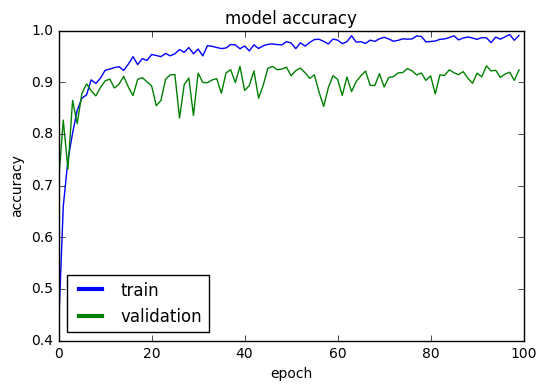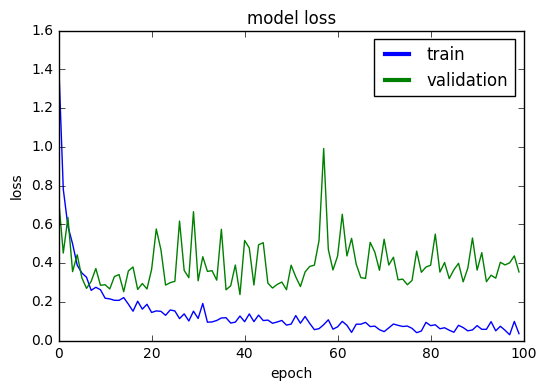In :
loss, accuracy = model.evaluate(X_train, Y_train, verbose=0)
print("Training: accuracy = %f  ;  loss = %f" % (accuracy, loss))

Training: accuracy = 0.998500  ;  loss = 0.009213

In :
loss, accuracy = model.evaluate(X_test, Y_test, verbose=0)
print("Validation: accuracy1 = %f  ;  loss1 = %f" % (accuracy, loss))

Validation: accuracy1 = 0.895625  ;  loss1 = 1.100054


Although the training accuracy is quite high (99.82% !), the overall result is not good. The 10% gap with the validation accuracy is an indication of overfitting (which is also clearly noticeable from the accuracy and loss graphs above). Our model is successful on the training set only and is no as successful for any other data.

## Inspecting the output¶

Befor we search for a new model, let's take a quick look on some of the cases that our model missed. It may give us clues on the strengths and weaknesses of NN models, and what we can expect from these artificial models.

The predict_classes method is helpful for getting a vector (y_pred) of the predicted classes of model1. We should compare y_pred to the expected true classes y_test in order to get the false cases:

In :
y_pred = model.predict_classes(X_test)

4992/5000 [============================>.] - ETA: 0s
In :
true_preds = [(x,y) for (x,y,p) in zip(X_test, y_test, y_pred) if y == p]
false_preds = [(x,y,p) for (x,y,p) in zip(X_test, y_test, y_pred) if y != p]
print("Number of valid predictions: ", len(true_preds))
print("Number of invalid predictions:", len(false_preds))

Number of valid predictions:  4618
Number of invalid predictions: 382


The array false_preds consists of all triples (x,y,p) where x is an image, y is its true class, and p is the false predicted value of model.

Lets visualize a sample of 15 items:

In :
for i,(x,y,p) in enumerate(false_preds[0:15]):
plt.subplot(3, 5, i+1)
plt.imshow(x, cmap='jet')
plt.title("%d\ny: %s\np: %s" % (i, class_name[y], class_name[p]), fontsize=9, loc='left')
plt.axis('off')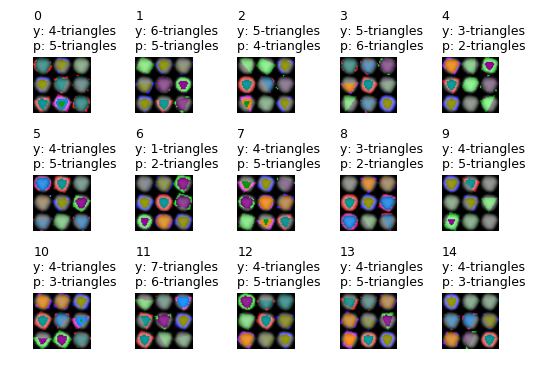We see that in all cases our model missed the correct answer by 1, which is almost like a human error.

## Second Keras Model for FigTab database¶

Lets try to add an additional Convolution2D layer and reduce the width of the Dense layers. The number of parameters is still too high (32 millions), but much less than model 1.

In :
nb_epoch = 100
batch_size = 32
input_shape = X_train.shape[1:]

model = Sequential(name="model_2")

print(model.summary())
save_model_summary(model, "model_2_summary.txt")
write_file("model_2.json", model.to_json())
fmon = FitMonitor(thresh=0.09, minacc=0.999, filename="model_2_autosave.h5")

hist = model.fit(
X_train,
Y_train,
batch_size=batch_size,
nb_epoch=nb_epoch,
shuffle=True,
validation_data=(X_test, Y_test),
verbose=0,
callbacks = [fmon]
)

model_file = "model_2.h5"
print("Saving model to:", model_file)
model.save(model_file)
plot(model, to_file="model_2_scheme.png", show_layer_names=False, show_shapes=True)

show_scores(model, hist, X_train, Y_train, X_test, Y_test)

____________________________________________________________________________________________________
Layer (type)                     Output Shape          Param #     Connected to
====================================================================================================
convolution2d_2 (Convolution2D)  (None, 46L, 46L, 64)  1792        convolution2d_input_2
____________________________________________________________________________________________________
srelu_4 (SReLU)                  (None, 46L, 46L, 64)  541696      convolution2d_2
____________________________________________________________________________________________________
convolution2d_3 (Convolution2D)  (None, 44L, 44L, 64)  36928       srelu_4
____________________________________________________________________________________________________
srelu_5 (SReLU)                  (None, 44L, 44L, 64)  495616      convolution2d_3
____________________________________________________________________________________________________
flatten_2 (Flatten)              (None, 123904)        0           srelu_5
____________________________________________________________________________________________________
dense_4 (Dense)                  (None, 256)           31719680    flatten_2
____________________________________________________________________________________________________
srelu_6 (SReLU)                  (None, 256)           1024        dense_4
____________________________________________________________________________________________________
dropout_3 (Dropout)              (None, 256)           0           srelu_6
____________________________________________________________________________________________________
dense_5 (Dense)                  (None, 64)            16448       dropout_3
____________________________________________________________________________________________________
srelu_7 (SReLU)                  (None, 64)            256         dense_5
____________________________________________________________________________________________________
dropout_4 (Dropout)              (None, 64)            0           srelu_7
____________________________________________________________________________________________________
dense_6 (Dense)                  (None, 10)            650         dropout_4
____________________________________________________________________________________________________
activation_2 (Activation)        (None, 10)            0           dense_6
====================================================================================================
Total params: 32,814,090
Trainable params: 32,814,090
Non-trainable params: 0
____________________________________________________________________________________________________
None
Train begin: 2017-01-06 10:04:08
Stop file: stop_training_file.keras (create this file to stop training gracefully)
Pause file: pause_training_file.keras (create this file to pause training and view graphs)
batch_size = 32
do_validation = True
metrics = ['loss', 'acc', 'val_loss', 'val_acc']
nb_epoch = 100
nb_sample = 10000
verbose = 0
.....05% epoch=5, acc=0.827500, loss=0.430133, val_acc=0.887600, val_loss=0.304704, time=0.136 hours
.....10% epoch=10, acc=0.914000, loss=0.239956, val_acc=0.924200, val_loss=0.199444, time=0.249 hours
.....15% epoch=15, acc=0.939600, loss=0.178788, val_acc=0.804800, val_loss=0.610535, time=0.362 hours
.....20% epoch=20, acc=0.951400, loss=0.152475, val_acc=0.940400, val_loss=0.199963, time=0.475 hours
.....25% epoch=25, acc=0.964300, loss=0.111787, val_acc=0.960800, val_loss=0.137968, time=0.588 hours
.....30% epoch=30, acc=0.961300, loss=0.126416, val_acc=0.951200, val_loss=0.162836, time=0.701 hours
.....35% epoch=35, acc=0.966500, loss=0.108984, val_acc=0.956400, val_loss=0.130644, time=0.814 hours
.....40% epoch=40, acc=0.972300, loss=0.105506, val_acc=0.920000, val_loss=0.231536, time=0.927 hours
.....45% epoch=45, acc=0.970700, loss=0.109364, val_acc=0.951200, val_loss=0.169184, time=1.040 hours
.....50% epoch=50, acc=0.977000, loss=0.074945, val_acc=0.966600, val_loss=0.110312, time=1.153 hours
.....55% epoch=55, acc=0.976600, loss=0.079870, val_acc=0.967200, val_loss=0.104487, time=1.265 hours
.....60% epoch=60, acc=0.980900, loss=0.066343, val_acc=0.901200, val_loss=0.405149, time=1.378 hours
.....65% epoch=65, acc=0.975500, loss=0.089095, val_acc=0.962600, val_loss=0.123806, time=1.491 hours
.....70% epoch=70, acc=0.973600, loss=0.099350, val_acc=0.964800, val_loss=0.124647, time=1.604 hours
.....75% epoch=75, acc=0.986500, loss=0.046769, val_acc=0.965600, val_loss=0.128774, time=1.717 hours
.....80% epoch=80, acc=0.985600, loss=0.049357, val_acc=0.955200, val_loss=0.166943, time=1.830 hours
.....85% epoch=85, acc=0.991500, loss=0.037036, val_acc=0.949400, val_loss=0.235901, time=1.942 hours
.....90% epoch=90, acc=0.979600, loss=0.067485, val_acc=0.966800, val_loss=0.118431, time=2.056 hours
.....95% epoch=95, acc=0.982800, loss=0.061920, val_acc=0.967000, val_loss=0.115121, time=2.169 hours
.... 99% epoch=99 acc=0.988700 loss=0.040717
Train end: 2017-01-06 12:19:44
Total run time: 2.260 hours
max_acc = 0.991500  epoch = 85
max_val_acc = 0.972200  epoch = 63
No checkpoint model found.
Saving model to: model_2.h5
Training: accuracy   = 0.998400 loss = 0.006470
Validation: accuracy = 0.950600 loss = 0.233240
Over fitting score   = 0.030267
Under fitting score  = 0.034055
Params count: 32814090
stop epoch = 99
nb_epoch = 100
batch_size = 32
nb_sample = 10000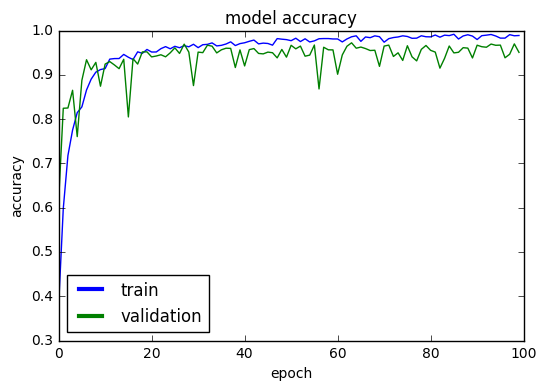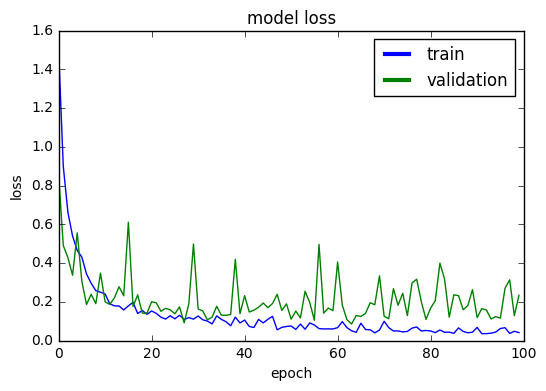Adding more Convolution layers has reduced overfitting from 10% to 5%, but this is not good enough yet. The gap between the training and validation loss graph indicates that there's more room for improvement.

## Validation credibility¶

Before proceeding to our third model, let's take a moment for discussing one more isuue. From the two models we learn that training accuracy can be quite high, but we should not be impressed as we fall short in our validation sets. In some cases however we might be satisfied with what we got but would like to carry out further tests to make sure that the validation accuracy we have is not volatile. After all our validation set ("test.h5") has only 5000 samples, which might not be enough to trust our model.

Our imgutils contains a special method check_data_set for testing our model on as many samples as we wish from our large repository of samples (320K samples!). This method accepts three arguments:

1. Keras model object
2. HDF5 file of FigTab images
3. Number of images to sample

You may want to sample a few thousand images from each repository in order to gain confidence in your model. here are two examples of using this method which show that the validation accuracy we got is trustable:

In :
check_data_set(model, "figtab1.h5")

Total num images in file: 50000
Time: 16.78 seconds
50000/50000 [==============================] - 37s
Data shape: (50000L, 48L, 48L, 3L)
accuracy   = 0.948360 loss = 0.258671

Out:
(0.94835999999999998, 0.25867102639141493)
In :
check_data_set(model, "figtab2.h5", sample=20000)

Total num images in file: 50000
Sampling 20000 images from 50000
Time: 7.29 seconds
20000/20000 [==============================] - 15s
Data shape: (20000L, 48L, 48L, 3L)
accuracy   = 0.948500 loss = 0.266648

Out:
(0.94850000000000001, 0.26664756789351812)

In both cases we get a validation score which pretty close to the one we got on our small validation data set.

## Model 3¶

We will add a third Convolution layer, and increase the filter size to 5x5 in the first two layers. In adition, we add three new MaxPooling2D layers (one after each Convolution2D). The immediate effect of these layers is a drastic reduction in the model number of parameters from 90 million to 915K almost 1% compared to model 1. Even if we get similar results to model 1, it would be considered a success and a proof for why Convolution and Pooling layers are the right kind of layers to use for image data.

In :
nb_epoch = 100
batch_size = 32
input_shape = X_train.shape[1:]

model = Sequential(name="model_3")

print(model.summary())
save_model_summary(model, "model_3_summary.txt")
write_file("model_3.json", model.to_json())
fmon = FitMonitor(thresh=0.09, minacc=0.999, filename="model_3_autosave.h5")

hist = model.fit(
X_train,
Y_train,
batch_size=batch_size,
nb_epoch=nb_epoch,
shuffle=True,
validation_data=(X_test, Y_test),
verbose=0,
callbacks = [fmon]
)

model_file = "model_3.h5"
print("Saving model to:", model_file)
model.save(model_file)
plot(model, to_file="model_3_scheme.png", show_layer_names=False, show_shapes=True)

show_scores(model, hist, X_train, Y_train, X_test, Y_test)

____________________________________________________________________________________________________
Layer (type)                     Output Shape          Param #     Connected to
====================================================================================================
convolution2d_4 (Convolution2D)  (None, 44L, 44L, 64)  4864        convolution2d_input_3
____________________________________________________________________________________________________
srelu_8 (SReLU)                  (None, 44L, 44L, 64)  495616      convolution2d_4
____________________________________________________________________________________________________
maxpooling2d_1 (MaxPooling2D)    (None, 22L, 22L, 64)  0           srelu_8
____________________________________________________________________________________________________
convolution2d_5 (Convolution2D)  (None, 18L, 18L, 64)  102464      maxpooling2d_1
____________________________________________________________________________________________________
srelu_9 (SReLU)                  (None, 18L, 18L, 64)  82944       convolution2d_5
____________________________________________________________________________________________________
maxpooling2d_2 (MaxPooling2D)    (None, 9L, 9L, 64)    0           srelu_9
____________________________________________________________________________________________________
convolution2d_6 (Convolution2D)  (None, 7L, 7L, 64)    36928       maxpooling2d_2
____________________________________________________________________________________________________
srelu_10 (SReLU)                 (None, 7L, 7L, 64)    12544       convolution2d_6
____________________________________________________________________________________________________
maxpooling2d_3 (MaxPooling2D)    (None, 3L, 3L, 64)    0           srelu_10
____________________________________________________________________________________________________
flatten_3 (Flatten)              (None, 576)           0           maxpooling2d_3
____________________________________________________________________________________________________
dense_7 (Dense)                  (None, 512)           295424      flatten_3
____________________________________________________________________________________________________
srelu_11 (SReLU)                 (None, 512)           2048        dense_7
____________________________________________________________________________________________________
dropout_5 (Dropout)              (None, 512)           0           srelu_11
____________________________________________________________________________________________________
dense_8 (Dense)                  (None, 256)           131328      dropout_5
____________________________________________________________________________________________________
srelu_12 (SReLU)                 (None, 256)           1024        dense_8
____________________________________________________________________________________________________
dropout_6 (Dropout)              (None, 256)           0           srelu_12
____________________________________________________________________________________________________
dense_9 (Dense)                  (None, 10)            2570        dropout_6
____________________________________________________________________________________________________
activation_3 (Activation)        (None, 10)            0           dense_9
====================================================================================================
Total params: 1,167,754
Trainable params: 1,167,754
Non-trainable params: 0
____________________________________________________________________________________________________
None
Train begin: 2017-01-06 12:33:06
Stop file: stop_training_file.keras (create this file to stop training gracefully)
Pause file: pause_training_file.keras (create this file to pause training and view graphs)
batch_size = 32
do_validation = True
metrics = ['loss', 'acc', 'val_loss', 'val_acc']
nb_epoch = 100
nb_sample = 10000
verbose = 0
.....05% epoch=5, acc=0.978000, loss=0.067423, val_acc=0.994400, val_loss=0.020790, time=0.034 hours
.....10% epoch=10, acc=0.980400, loss=0.068991, val_acc=0.981600, val_loss=0.065989, time=0.062 hours
.....15% epoch=15, acc=0.988900, loss=0.039010, val_acc=0.999400, val_loss=0.001800, time=0.090 hours
.....20% epoch=20, acc=0.977800, loss=0.084178, val_acc=0.999800, val_loss=0.000614, time=0.119 hours
.....25% epoch=25, acc=0.993000, loss=0.024323, val_acc=1.000000, val_loss=0.000051, time=0.147 hours
...
Saving model to model_3_autosave.h5: epoch=28, acc=0.999900, val_acc=1.000000
..30% epoch=30, acc=0.977900, loss=0.096000, val_acc=0.999800, val_loss=0.000273, time=0.176 hours
.....35% epoch=35, acc=0.993600, loss=0.026081, val_acc=1.000000, val_loss=0.000180, time=0.205 hours
..
Saving model to model_3_autosave.h5: epoch=37, acc=1.000000, val_acc=1.000000
...40% epoch=40, acc=0.992000, loss=0.037880, val_acc=0.999400, val_loss=0.001145, time=0.233 hours
.....45% epoch=45, acc=0.988300, loss=0.060651, val_acc=1.000000, val_loss=0.000031, time=0.262 hours
.....50% epoch=50, acc=0.996700, loss=0.015444, val_acc=1.000000, val_loss=0.000039, time=0.290 hours
.....55% epoch=55, acc=0.990100, loss=0.041218, val_acc=1.000000, val_loss=0.000017, time=0.318 hours
.....60% epoch=60, acc=0.989800, loss=0.046525, val_acc=0.999800, val_loss=0.001978, time=0.347 hours
.....65% epoch=65, acc=0.999400, loss=0.002954, val_acc=1.000000, val_loss=0.000036, time=0.375 hours
.....70% epoch=70, acc=0.989900, loss=0.045159, val_acc=1.000000, val_loss=0.000019, time=0.404 hours
Train end: 2017-01-06 12:57:19
Total run time: 0.404 hours
max_acc = 1.000000  epoch = 37
max_val_acc = 1.000000  epoch = 11
Best model saved in file: model_3_autosave.h5
Checkpoint: epoch=37, acc=1.000000, val_acc=1.000000
Saving model to: model_3.h5
Training: accuracy   = 1.000000 loss = 0.000004
Validation: accuracy = 1.000000 loss = 0.000019
Over fitting score   = 0.008188
Under fitting score  = 0.019507
Params count: 1167754
stop epoch = 70
nb_epoch = 100
batch_size = 32
nb_sample = 10000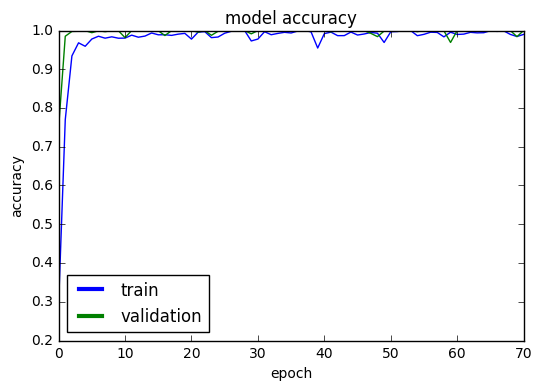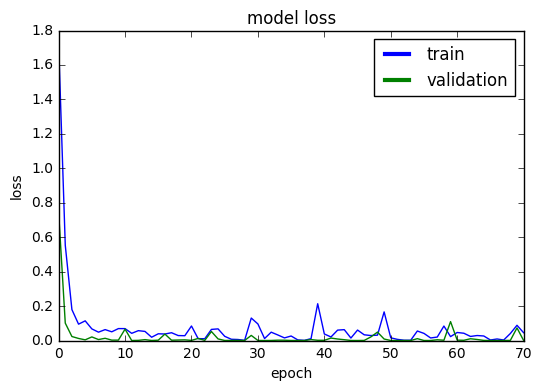In :
loss, accuracy = model.evaluate(X_train, Y_train, verbose=0)
print("Training: accuracy = %f  ;  loss = %f" % (accuracy, loss))

Training: accuracy = 1.000000  ;  loss = 0.000004

In :
loss, accuracy = model.evaluate(X_test, Y_test, verbose=0)
print("Validation: accuracy = %f  ;  loss = %f" % (accuracy, loss))

Validation: accuracy = 1.000000  ;  loss = 0.000019


This is one of the very rare occasions in which our neural network reached 100% training and validation accuracy! The second important point to bear in mind is that the number of parameters in our neural network has dropped from 70 million to 1 million! It means that with 1/70 of the size of our first model we were able to do much better.

This undoutably supports the claim that convolution and pooling layers are better fit for image processing than usual Dense layers.

To gain full confidence in our model, we will test it on our two large data sets figtab1.h5 and figtab2.h5 which contain 100,000 new samples.

In :
check_data_set(model, "figtab1.h5")

Total num images in file: 50000
Time: 16.38 seconds
49984/50000 [============================>.] - ETA: 0sData shape: (50000L, 48L, 48L, 3L)
accuracy   = 0.999840 loss = 0.001523

Out:
(0.99983999999999995, 0.0015229540972909671)
In :
check_data_set(model, "figtab2.h5")

Total num images in file: 50000
Time: 16.55 seconds
49984/50000 [============================>.] - ETA: 0sData shape: (50000L, 48L, 48L, 3L)
accuracy   = 0.999960 loss = 0.000308

Out:
(0.99995999999999996, 0.00030779796368726238)

Indeed, in both cases our model is achieving a very high accuracy score: 99.984% and 99.996%. This is a strong evidence for the validty and usefullness of our model when we apply it on new unknown samples.

Let's take a look on some of very few cases in which it fails.

In :
X1, y1, Y1, X2, y2, Y2 = load_data('figtab1.h5', 'figtab2.h5')
y_pred = model.predict_classes(X1)

Loading training data set: figtab1.h5
Total num images in file: 50000
Time: 16.54 seconds
Total num images in file: 50000
Time: 16.50 seconds
50000 training samples
50000 validation samples
Image shape: (48L, 48L, 3L)
49984/50000 [============================>.] - ETA: 0s
In :
true_preds = [(x,y) for (x,y,p) in zip(X1, y1, y_pred) if y == p]
false_preds = [(x,y,p) for (x,y,p) in zip(X1, y1, y_pred) if y != p]
print("Number of valid predictions: ", len(true_preds))
print("Number of invalid predictions:", len(false_preds))

Number of valid predictions:  49992
Number of invalid predictions: 8


We have only 8 failureד from 50000 samples! Let's draw them all:

In :
for i,(x,y,p) in enumerate(false_preds):
plt.subplot(2, 4, i+1)
plt.imshow(x, cmap='jet')
plt.title("%d\ny: %s\np: %s" % (i, class_name[y], class_name[p]), fontsize=9, loc='left')